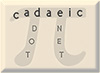Keith Numbers Mike Keith

A Keith Number is an n-digit integer N with the following property: If a Fibonacci-like sequence (in which each term in the sequence is the sum of the n previous terms) is formed, with the first n terms being the decimal digits of the number N, then N itself occurs as a term in the sequence. For example, 197 is a Keith number since it generates the sequence

1, 9, 7, 17, 33, 57, 107, 197, ...

Keith numbers are listed in Neil Sloane's On-Line Encyclopedia of Integer Sequences (look at sequence #7629) and at Wolfram MathWorld (just search for "Keith number"). I introduced them in a paper in 1987 (where they were called repfigit numbers or repfigits) and they proved popular enough to inspire several papers by other authors, most notably a whole series of papers that appeared in 1994 in Volume 26, Number 3 of the Journal of Recreational Mathematics.

These numbers are intruiging for several reasons. Firstly, they are very hard to find, since finding all Keith numbers with n digits is equivalent to solving a set of linear Diophantine equations with n variables.  In general, solving Diophantine equations is as hard as solving an n-element knapsack problem, which is NP-complete.  It could be the case that the specific Diophantine equations involved here can be solved using some technique which is not NP-hard, but no such technique is known. As a result, the existing algorithms for finding all Keith numbers with n digits have a run time that grows faster than linearly as n increases.

These numbers are in some ways reminscent of the primes in their unpredictable appearance among the integers. However, they are much rarer than the primes, with only a handful appearing between each power of 10.

Here is the complete list of Keith numbers less than 1029:

 No. Digits Keith Numbers 2 14 19 28 47 61 75 3 197 742 4 1104 1537 2208 2580 3684 4788 7385 7647 7909 5 31331 34285 34348 55604 62662 86935 93993 6 120284 129106 147640 156146 174680 183186 298320 355419 694280 925993 7 1084051 7913837 8 11436171 33445755 44121607 9 129572008 251133297 10 (none) 11 24769286411 96189170155 12 171570159070 202366307758 239143607789 296658839738 13 1934197506555 8756963649152 14 43520999798747 74596893730427 97295849958669 15 120984833091531 270585509032586 754788753590897 16 3621344088074041 3756915124022254 4362827422508274 17 11812665388886672 14508137312404344 16402582054271374 69953250322018194 73583709853303061 18 119115440241433462 166308721919462318 301273478581322148 19 1362353777290081176 3389041747878384662 5710594497265802190 5776750370944624064 6195637556095764016 20 12763314479461384279  27847652577905793413 45419266414495601903 21 855191324330802397989 22 7657230882259548723593 23 26842994422637112523337  36899277593852609997403 61333853602129819189668 24 229146413136585558461227 25 9838678687915198599200604 26 18354972585225358067718266 19876234926457288511947945 98938191214220718050301312 27 133118411174059688391045955 153669354455482560987178342 154140275428339949899922650 154677881401007799974564336 295768237361291708645227474 956633720464114515890318410 988242310393860390066911414 28 9493976840390265868522067200 29 41796205765147426974704791528 70267375510207885242218837404

Those up to 15 digits were published in the aforementioned issue of J. Rec. Math. I discovered the 16-, 17-, 18- and 19-digit specimens in Sept/Oct, 1998 using a slightly improved exhaustive search scheme.  Those 20 digits and longer were found in 2004 by Daniel Lichtblau of Wolfram Research employing a powerful approach involving the use of integer linear programming to solve the relevant Diophantine equations.

The number shown in red in the table above, discovered in 2004 by D. Lichtblau, finally answers a challenge I posed in 1998, which was to find the smallest pandigital Keith number (containing each of the digits 0 to 9 at least once).

The sequence of prime Keith numbers begins:

19, 47, 61, 197, 1084051, 74596893730427, ...

Note that all those having 25 digits or more end with a digit (0,2,4,6,8, or 5) which immediately tells us they are not prime.  It is not known if this is a long-term trend - if so, then we have found the largest prime Keith number already.

The number of Keith numbers with d digits (for d=2, 3, ..., 29) is

6, 2, 9, 7, 10, 2, 3, 2, 0, 2, 4, 2, 3, 3, 3, 5, 3, 5, 3, 1, 1, 3, 1, 1, 3, 7, 2, 1...

for a total of 94 less than 1029.

There are still a number of unanswered questions about these numbers, such as::

1. Are there an infinite number of Keith numbers? Heuristic arguments, and the numerical evidence above, both strongly suggest that the answer is "yes" - in fact, we expect to find roughly (9/10)log210 (about 3) of them between each power of 10. But there is still no proof, constructive or otherwise, that there are an infinite number of them.
2. Define a cluster of Keith numbers as a set of two or more (all with the same number of digits) in which all the numbers are integer multiples of the smallest one in the set. There are only three known clusters: (14, 28), (1104, 2208), and the remarkable - for having three members - (31331, 62662, 93993). Question: is the number of Keith clusters finite or infinite? Not only do we conjecture it is finite, but we conjecture that the above three clusters are the only ones.  But we have no clue how to prove this.
3. Is n=10 the only number of digits for which there are no Keith numbers?  (We tentatively think not, but it may be a while before another one is found.)

Since we suspect that they go on forever, the problem of finding the next such number in the exhaustive list, or isolated larger examples, always remains a tantalizing one.

Finally, note that these can be generalized so we do the computations in base b. I have an unpublished manuscript by Kenneth Fan that details how to construct all Keith numbers (of which there are an infinite number) in base 2; so, in effect, the problem is solved for the binary case. It may be that base 2 is the only "easy" base, however - certainly their properties in base 10 remain quite mysterious.